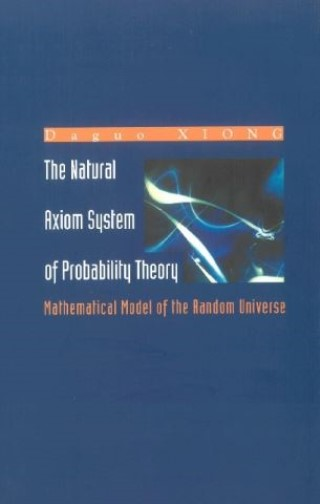## Natural Axiom System Of Probability Theory, The: Mathematical Model Of The Random Universe• Description
• Author
• Info
• Reviews

### Description

The causation space established in this book is a mathematical model of the random universe and a “living house” of all random tests and probability spaces. By using this space, one can introduce the mathematical calculation methods related to probability spaces and random tests. The book also points out that the basic unit to be studied in the probability theory is the random test, and not a stand-alone event.